Most Affordable JEE | NEET | 8,9,10 Preparation by Kota's Top IITian Doctor Faculties

# What is Conduction - Explanation, Definition - eSaral## What is Conduction

Conduction is that mode of transmission of heat in which heat is transferred from a region of higher temperature to a region of lower temperature by the aid of particles of the body without their actual migration.

In conduction atoms or molecules near the hot end absorb energy and hence their amplitude of vibration about their mean position increases due to which they collide with other neighbouring atoms or molecules and thus transfer a part of energy, to them and so on. In solids, heat transfer is mainly by conduction.

In metals the conduction of heat takes place by means of free electrons present in the metal.

When one end of a rod is heated, then initially the temperature of various points of the rod changes continuously and the rod is said to exist in a variable state. After some time, a state is reached, when the temperature of each cross-section becomes steady. This state is known as steady state. In this state, any heat received by any cross-section is partly conducted to the next section and partly radiated, i.e., no heat is absorbed by the cross-section.

By steady state it does not, imply that the temperature of the whole rod is same. In steady state, the temperature of the different parts of the rod are different but temperature of the each part remains constant. The temperature decreases, as we move away from the hot end.

In this state rate of heat flow depends only on the heat conductivity of the material of the rod so it remains constant.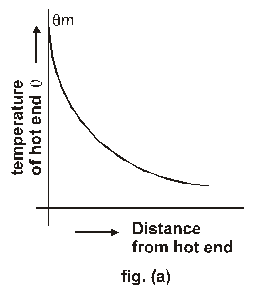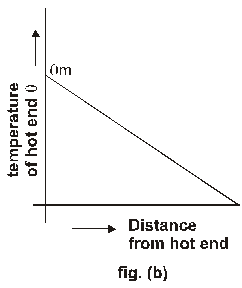If rod is in contact with atmosphere or surroundings and it is heated at one end then distance versus temperature curve is as shown in fig. (a)

And if rod is insultated with surroundings then graph is stright line as shown in fig. (b)

Isothermal Surface In steady state if heat loss due to radiation or convection in negligible then the temperature of of every transverse section of the rod is same on the whole area of section (Because the cross-section is perfecdicular to the direction of flow of heat hence there will be no heat flow from one point to other on the cross-section). Thus every transverse section of the rod behaves as on Isothermal surface.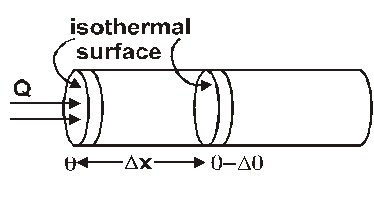The rate of change of temperature with distance between two isothermal surfaces is called temperature gradiant.

If the temperature of the isothermal surfaces be q and q – Dq and perpendicular distance between then in Dx

$=\frac{\text { change in temperature }}{\text { distan ce }}=\frac{(\theta-\Delta \theta)-\theta}{\Delta \mathrm{x}}=-\frac{\Delta \theta}{\Delta \mathrm{x}}$

The negative singn show that temperture q decreases as the distance x increases in the directin of heat flow.

Unit: ºC/meter

## Thermal Conductivity

If a steady temperature difference (q1 – q2) is to be maintained between the ends of a rod, heat must be supplied at a steady rate at one end and the same must be taken out at the other end. Suppose an amount of heat Q flows through the rod in time t

then rate of heat flow or Heat current $\mathrm{H}=\frac{\mathrm{Q}}{\mathrm{t}}$

It has been found experimentally that in steady state, Heat current $H=\frac{Q}{t}$ will be
1. Proportional to the area A of the cross-section of the rod. The larger the cross-sectional area, the greater will be the number of molecules that conduct heat via collisions.
2. Proportional to (q1 – q2). The greater the temperature difference to be maintained, the greater must be the rate of heat flow.
3. Inversely proportional to l, the distance between ends of the rod. The smaller the distance l, the greater must be the rate of heat flow to maintain a given temperature difference.
Thus we find that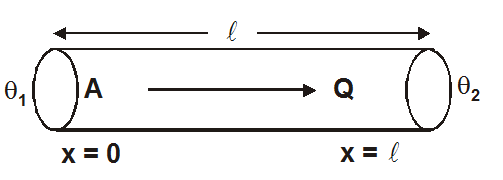$\frac{\mathrm{Q}}{\mathrm{t}} \propto \frac{\mathrm{A}\left(\theta_{1}-\theta_{2}\right)}{\ell}$ or $\mathrm{H}=\frac{\mathrm{KA}\left(\theta_{1}-\theta_{2}\right)}{\ell}$

where K is a constant of proportionality called the thermal conductivity of the substance. It is a measure of how quickly heat energy can conduct (flow) through the substance.

## Coefficient of thermal conductivity (K) :

The coefficient of thermal conductivity, K, of a material is defined as the amount of heat that flowing per second through a rod of that material of having unit length and unit area of cross-section in the steady state, when the difference of temperature between two ends of the rod is 1 ºC and flow of heat is perpendicular to the end faces of the rod.

Unit of K : CGS = cal/cm-sec-°C MKS = kcal/m-sec-K SI = watt/m-K

DIMENSIONS : $\left[M L T^{-3} \theta^{-1}\right]$

For a perfect conductor of heat K is infinite and for a perfect insulator K is zero.

In general, solids are better conductors than liquids and liquids are better conductors than gases. (Heat transfer through process of conduction is possible in liquids and gases also, if they are heated from the top.) Metals are much better conductors than non-metals.

a good conductor of heat is also a good conductor of electricity
The conduction of both heat and electricity is due to the movement of free electrons. Wiedeman and Franz established a relation between thermal conductivity (K) and electrical conductivity s().

## Thermal Resistance :

The thermal resistance of a body is a measure of its opposition to the flow of heat through it.
It is defined as the ratio of temperature difference to the heat current (= rate of flow of heat)

Now, temperature difference = (q1 ~ q2)

l = Length of rod

heat current, $\mathrm{H}=\frac{\mathrm{Q}}{\mathrm{t}}$

A = Area of cross section of rod

$\mid \mathrm{R}_{\text {Th }}=\frac{\theta_{1} \sim \theta_{2}}{\mathrm{H}}=\frac{\theta_{1} \sim \theta_{2}}{(\mathrm{Q} / \mathrm{t})}=\frac{\theta_{1} \sim \theta_{2}}{\mathrm{KA}\left(\theta_{1} \sim \theta_{2}\right) / \ell}=\frac{\ell}{\mathrm{KA}}$

or $\mid R_{T h}=\frac{\Delta \theta}{H}=\frac{\ell}{K A}$

Unit : °C × sec/cal or K × sec/k-calorie.

dimensons : $\left[M^{-1} \quad L^{-2} T^{3} \theta\right]$

Ex. A wall has two layers A and B, each made of a different material, Both the layers have the same thickness. The thermal conductivity of the material of A is twice that of B. Under thermal equilibrium, the temperature difference across the wall is 36°C. The temperature difference across the layer A.

Sol: Let $\Delta \theta_{1}$ and $\Delta \theta_{2}$ be the temperature differences across layers $A$ and $B$. In series rate of flow of heat is same $\mathrm{H}_{1}=\mathrm{H}_{2}$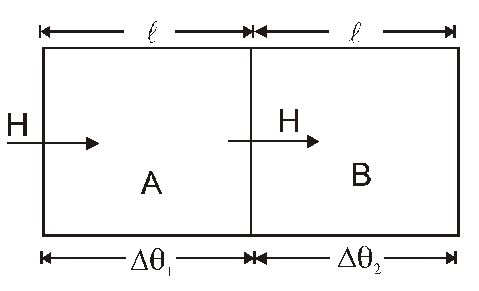$\frac{\mathrm{K}_{1}\left(\Delta \theta_{1}\right)}{\ell}=\frac{\mathrm{K}_{2}\left(\Delta \theta_{2}\right)}{\ell}$

i.e. $\frac{\Delta \theta_{1}}{\Delta \theta_{2}}=\frac{\mathrm{K}_{2}}{\mathrm{~K}_{1}}$

Given $\mathrm{K}_{1}=2 \mathrm{~K}_{2} \quad \therefore \quad \frac{\Delta \theta_{1}}{\Delta \theta_{2}}=\frac{\mathrm{K}_{2}}{\mathrm{~K}_{1}}=\frac{1}{2}$

or $\quad \Delta \theta_{2}=2 \Delta \theta_{1}$

Also in series $\Delta \theta_{1}+\Delta \theta_{2}=36^{\circ}$

$\Delta \theta_{1}+2 \Delta \theta_{1}=36^{\circ}$

$3 \Delta \theta_{1}=36^{\circ}$

$\Delta \theta_{1}=\frac{36}{3}=12^{\circ} \mathrm{C}$

For a better understanding of this chapter, please check the detailed notes of the Thermodynamics. If you want more Free Learning Videos and Study Material Then don't forget to download the eSaral App.